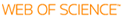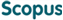### BROWSE

A Finite-Difference Time-Domain simulation of Laser Absorption in Metals

Cited 0 times inCited 0 times inTitle
A Finite-Difference Time-Domain simulation of Laser Absorption in Metals
Author
Issue Date
2013-08
Publisher
Abstract
Laser beam is an electromagnetic (EM) wave, which means that it is governed by Maxwell’s equations. In this study, we adopted the finite-difference time-domain (FDTD) method, to fully solve the Maxwell’s equations to obtain the accurate description of laser propagation. Since the focus is laser interaction with materials, so it’s very important to define a certain kind of material in the computational domain. In general there are 2 types of materials, dielectrics like glass and dispersive materials like metal. For calculating the beam absorption in materials: 1)Because of the dispersive characteristic of dispersive material, the standard FDTD is no longer suitable and not be able to describe the material behavior. Thus, 2 different codes for different materials have to be used, which loses the generality. In this study, a method of simulating dispersive materials by changing refractive index and extinction number of the material is discussed. In this regard, it is possible to use only one simple standard FDTD code to simulate a general material, no matter it’s dielectric or dispersive. 2) Laser simulation always prefers a larger scale problem but this is extremely difficult because the small wavelength results in the extremely high burden of the computers. A method of enlarge the wavelength while keeping the same reflectance is developed in this study, thus a larger domain can be simulated. The simulations were conducted in 3 dimensions and perfectly matched layer (PML) absorption boundary condition was employed to terminate the computational domain, avoiding the numerically generated artificial waves. Reflectance of different polarizations (P-polarization, S-polarization, Circular polarization) by computing the reflected energy flux using Poynting vector are computed with both standard and dispersive code, and the results proved the correctness of those methods. Absortance of a keyhole (tilted cylindrical hole) was calculated as an example.
Description
Mechanical Engineering
URI